Documentation

## Expected Shortfall Estimation and Backtesting

This example shows how to perform estimation and backtesting of Expected Shortfall models.

Value-at-Risk (VaR) and Expected Shortfall (ES) must be estimated together because the ES estimate depends on the VaR estimate. Using historical data, this example estimates VaR and ES over a test window, using historical and parametric VaR approaches. The parametric VaR is calculated under the assumption of normal and t distributions.

This example runs the ES back tests supported in the `esbacktest` and `esbacktestbysim` functionality to assess the performance of the ES models in the test window.

The `esbacktest` object does not require any distribution information. Like the `varbacktest` object, the `esbacktest` object only takes test data as input. The inputs to `esbacktest` include portfolio data, VaR data and corresponding VaR level, and also the ES data, since this is what is back tested. Like `varbacktest`, `esbacktest` runs tests for a single portfolio, but can back test multiple models and multiple VaR levels at once. The `esbacktest` object uses precomputed tables of critical values to determine if the models should be rejected. These table-based tests can be applied as approximate tests for any VaR model. In this example, they are applied to back test historical and parametric VaR models. They could be used for other VaR approaches such as Monte-Carlo or Extreme-Value models.

In contrast, the `esbacktestbysim` object requires the distribution information, namely, the distribution name (`normal` or `t`) and the distribution parameters for each day in the test window. `esbacktestbysim` can only back test one model at a time, because it is linked to a particular distribution, although it can still back test multiple VaR levels at once. The `esbacktestbysim` object implements simulation-based tests, and it uses the provided distribution information to run simulations to determine critical values. The `esbacktestbysim` object supports normal and t distributions. These tests can be used for any model where the underlying distribution of portfolio outcomes is normal or t, such as exponentially weighted moving average (EWMA), delta-gamma, or generalized autoregressive conditional heteroskedasticity (GARCH) models.

For additional information on the ES backtesting methodology, see `esbacktest` and `esbacktestbysim`, or see  in the References.

### Estimate VaR and ES

The data set used in this example contains historical data for the S&P index spanning approximately 10 years, from the middle of 1993 through the middle of 2003. The estimation window size is defined as 250 days, so that a full year of data is used to estimate both the historical VaR, and the volatility. The test window in this example runs from the beginning of 1995 through the end of 2002.

Throughout this example, a VaR confidence level of 97.5% is used, as required by the Fundamental Review of the Trading Book (FRTB) regulation; see .

```load VaRExampleData.mat Returns = tick2ret(sp); DateReturns = dates(2:end); SampleSize = length(Returns); TestWindowStart = find(year(DateReturns)==1995,1); TestWindowEnd = find(year(DateReturns)==2002,1,'last'); TestWindow = TestWindowStart:TestWindowEnd; EstimationWindowSize = 250; DatesTest = DateReturns(TestWindow); ReturnsTest = Returns(TestWindow); VaRLevel = 0.975;```

The historical VaR is a non-parametric approach to estimate the VaR and ES from historical data over an estimation window. The VaR is a percentile, and there are alternative ways to estimate the percentile of a distribution based on a finite sample. One common approach is to use the `prctile` function. An alternative approach is to sort the data and determine a cut point based on the sample size and VaR confidence level. Similarly, there are alternative approaches to estimate the ES based on a finite sample.

The `hHistoricalVaRES` local function on the bottom of this example uses a finite-sample approach for the estimation of VaR and ES following the methodology described in . In a finite sample, the number of observations below the VaR may not match the total tail probability corresponding to the VaR level. For example, for 100 observations and a VaR level of 97.5%, the tail observations are 2, which is 2% of the sample, however the desired tail probability is 2.5%. It could be even worse for samples with repeated observed values, for example, if the second and third sorted values were the same, both equal to the VaR, then only the smallest observed value in the sample would have a value less than the VaR, and that is 1% of the sample, not the desired 2.5%. The method implemented in `hHistoricalVaRES` makes a correction so that the tail probability is always consistent with the VaR level; see  for details.

```VaR_Hist = zeros(length(TestWindow),1); ES_Hist = zeros(length(TestWindow),1); for t = TestWindow i = t - TestWindowStart + 1; EstimationWindow = t-EstimationWindowSize:t-1; [VaR_Hist(i),ES_Hist(i)] = hHistoricalVaRES(Returns(EstimationWindow),VaRLevel); end```

The following plot shows the daily returns, and the VaR and ES estimated with the historical method.

```figure; plot(DatesTest,ReturnsTest,DatesTest,-VaR_Hist,DatesTest,-ES_Hist) legend('Returns','VaR','ES','Location','southeast') title('Historical VaR and ES') grid on```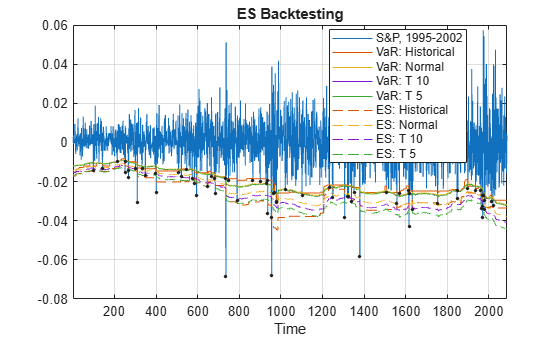For the parametric models, the volatility of the returns must be computed. Given the volatility, the VaR, and ES can be computed analytically.

A zero mean is assumed in this example, but can be estimated in a similar way.

For the normal distribution, the estimated volatility is used directly to get the VaR and ES. For the t location-scale distribution, the scale parameter is computed from the estimated volatility and the degrees of freedom.

The `hNormalVaRES` and `hTVaRES` local functions take as inputs the distribution parameters (which can be passed as arrays), and return the VaR and ES. These local functions use the analytical expressions for VaR and ES for normal and t location-scale distributions, respectively; see  for details.

```% Estimate volatility over the test window Volatility = zeros(length(TestWindow),1); for t = TestWindow i = t - TestWindowStart + 1; EstimationWindow = t-EstimationWindowSize:t-1; Volatility(i) = std(Returns(EstimationWindow)); end % Mu=0 in this example Mu = 0; % Sigma (standard deviation parameter) for normal distribution = Volatility SigmaNormal = Volatility; % Sigma (scale parameter) for t distribution = Volatility * sqrt((DoF-2)/DoF) SigmaT10 = Volatility*sqrt((10-2)/10); SigmaT5 = Volatility*sqrt((5-2)/5); % Estimate VaR and ES, normal [VaR_Normal,ES_Normal] = hNormalVaRES(Mu,SigmaNormal,VaRLevel); % Estimate VaR and ES, t with 10 and 5 degrees of freedom [VaR_T10,ES_T10] = hTVaRES(10,Mu,SigmaT10,VaRLevel); [VaR_T5,ES_T5] = hTVaRES(5,Mu,SigmaT5,VaRLevel); ```

The following plot shows the daily returns, and the VaR and ES estimated with the normal method.

```figure; plot(DatesTest,ReturnsTest,DatesTest,-VaR_Normal,DatesTest,-ES_Normal) legend('Returns','VaR','ES','Location','southeast') title('Normal VaR and ES') grid on```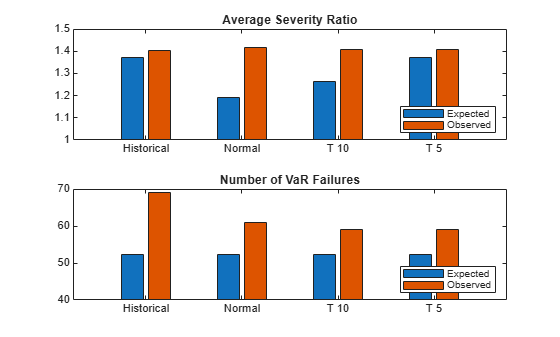For the parametric approach, the same steps can be used to estimate the VaR and ES for alternative approaches, such as EWMA, delta-gamma approximations, and GARCH models. In all these parametric approaches, a volatility is estimated every day, either from an EWMA update, from a delta-gamma approximation, or as the conditional volatility of a GARCH model. The volatility can then be used as above to get the VaR and ES estimates for either normal or t location-scale distributions.

### ES Backtest Without Distribution Information

The `esbacktest` object offers two back tests for ES models. Both tests use the unconditional test statistic proposed in , given by

`${\mathit{Z}}_{\mathrm{uncond}}=\frac{1}{{\mathrm{Np}}_{\mathrm{VaR}}}{\sum }_{\mathit{t}=1}^{\mathit{N}}\frac{{\mathit{X}}_{\mathit{t}}{\mathit{I}}_{\mathit{t}}}{{\mathrm{ES}}_{\mathit{t}}}+1$`

where

• $\mathit{N}$ is the number of time periods in the test window.

• ${\mathit{X}}_{\mathit{t}}$ is the portfolio outcome, that is, the portfolio return or portfolio profit and loss for period t.

• ${\mathit{p}}_{\mathrm{VaR}}$ is the probability of VaR failure defined as 1-VaR level.

• ${\mathrm{ES}}_{\mathit{t}}$ is the estimated expected shortfall for period t.

• ${\mathit{I}}_{\mathit{t}}$ is the VaR failure indicator on period t with a value of 1 if ${\mathit{X}}_{\mathit{t}}<{-\mathrm{VaR}}_{\mathit{t}}$, and 0 otherwise.

The expected value for this test statistic is 0, and it is negative when there is evidence of risk underestimation. To determine how negative it should be to reject the model, critical values are needed, and to determine critical values, distributional assumptions are needed for the portfolio outcomes ${\mathit{X}}_{\mathit{t}}$.

The unconditional test statistic turns out to be stable across a range of distributional assumptions for ${\mathit{X}}_{\mathit{t}}$, from thin-tailed distributions such as normal, to heavy-tailed distributions such as t with low degrees of freedom (high single digits). Only the most heavy-tailed t distributions (low single digits) lead to more noticeable differences in the critical values. See  for details.

The `esbacktest` object takes advantage of the stability of the critical values of the unconditional test statistic, and uses tables of precomputed critical values to run ES back tests. `esbacktest` has two sets of critical-value tables. The first set of critical values assumes that the portfolio outcomes ${\mathit{X}}_{\mathit{t}}$ follow a standard normal distribution; this is the `unconditionalNormal` test. The second set of critical values uses the heaviest possible tails, it assumes that the portfolio outcomes ${\mathit{X}}_{\mathit{t}}$ follow a t distribution with 3 degrees of freedom; this is the `unconditionalT` test.

The unconditional test statistic is sensitive to both the severity of the VaR failures relative to the ES estimate, and also to the number of VaR failures (how many times the VaR is violated). Therefore, a single but very large VaR failure relative to the ES (or only very few large losses) may cause the rejection of a model in a particular time window. A large loss on a day when the ES estimate is also large may not impact the test results as much as a large loss when the ES is smaller. And a model can also be rejected in periods with many VaR failures, even if all the VaR violations are relatively small and only slightly higher than the VaR. Both situations are illustrated in this example.

The `esbacktest` object takes as input the test data, but no distribution information is provided to `esbacktest`. Optionally, you can specify ID's for the portfolio, and for each of the VaR and ES models being backtested. Although the model ID's in this example do have distribution references (for example, `"normal"` or `"t 10"`), these are only labels used for reporting purposes. The tests do not use the fact that the first model is a historical VaR method, or that the other models are alternative parametric VaR models. The distribution parameters used to estimate the VaR and ES in the previous section are not passed to `esbacktest`, and are not used in any way in this section. These parameters, however, must be provided for the simulation-based tests supported in the `esbacktestbysim` object discussed in the Simulation-Based Tests section.

```ebt = esbacktest(ReturnsTest,[VaR_Hist VaR_Normal VaR_T10 VaR_T5],... [ES_Hist ES_Normal ES_T10 ES_T5],'PortfolioID',"S&P, 1995-2002",... 'VaRID',["Historical" "Normal","T 10","T 5"],'VaRLevel',VaRLevel); disp(ebt)```
``` esbacktest with properties: PortfolioData: [2087x1 double] VaRData: [2087x4 double] ESData: [2087x4 double] PortfolioID: "S&P, 1995-2002" VaRID: ["Historical" "Normal" "T 10" "T 5"] VaRLevel: [0.9750 0.9750 0.9750 0.9750] ```

Start the analysis by running the `summary` function.

```s = summary(ebt); disp(s)```
``` PortfolioID VaRID VaRLevel ObservedLevel ExpectedSeverity ObservedSeverity Observations Failures Expected Ratio Missing ________________ ____________ ________ _____________ ________________ ________________ ____________ ________ ________ ______ _______ "S&P, 1995-2002" "Historical" 0.975 0.96694 1.3711 1.4039 2087 69 52.175 1.3225 0 "S&P, 1995-2002" "Normal" 0.975 0.97077 1.1928 1.416 2087 61 52.175 1.1691 0 "S&P, 1995-2002" "T 10" 0.975 0.97173 1.2652 1.4063 2087 59 52.175 1.1308 0 "S&P, 1995-2002" "T 5" 0.975 0.97173 1.37 1.4075 2087 59 52.175 1.1308 0 ```

The `ObservedSeverity` column shows the average ratio of loss to VaR on periods when the VaR was violated. The `ExpectedSeverity` column uses the average ratio of ES to VaR for the VaR violation periods. For the `"Historical"` and `"T 5"` models, the observed and expected severities are comparable. However, for the `"Historical"` method, the observed number of failures (`Failures` column) is considerably higher than the expected number of failures (`Expected` column), about 32% higher (see the `Ratio` column). Both the `"Normal"` and the` "T 10"` models have observed severities much higher than the expected severities.

```figure; subplot(2,1,1) bar(categorical(s.VaRID),[s.ExpectedSeverity,s.ObservedSeverity]) ylim([1 1.5]) legend('Expected','Observed','Location','southeast') title('Average Severity Ratio') subplot(2,1,2) bar(categorical(s.VaRID),[s.Expected,s.Failures]) ylim([40 70]) legend('Expected','Observed','Location','southeast') title('Number of VaR Failures')```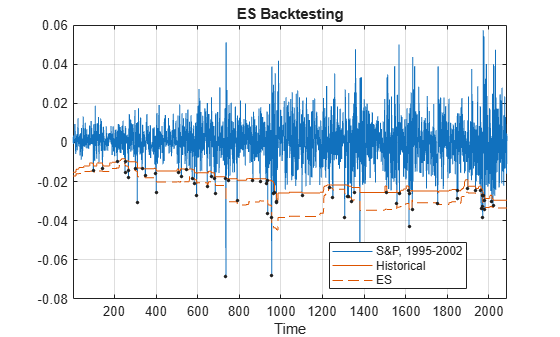The `runtests` function runs all tests and reports only the accept or reject result. The unconditional normal test is more strict. For the 8-year test window here, two models fail both tests (`"Historical"` and `"Normal"`), one model fails the unconditional normal test, but passes the unconditional t test (`"T 10"`), and one model passes both tests (`"T 5"`).

```t = runtests(ebt); disp(t)```
``` PortfolioID VaRID VaRLevel UnconditionalNormal UnconditionalT ________________ ____________ ________ ___________________ ______________ "S&P, 1995-2002" "Historical" 0.975 reject reject "S&P, 1995-2002" "Normal" 0.975 reject reject "S&P, 1995-2002" "T 10" 0.975 reject accept "S&P, 1995-2002" "T 5" 0.975 accept accept ```

Additional details on the tests can be obtained by calling the individual test functions. Here are the details for the `unconditionalNormal` test.

```t = unconditionalNormal(ebt); disp(t)```
``` PortfolioID VaRID VaRLevel UnconditionalNormal PValue TestStatistic CriticalValue Observations TestLevel ________________ ____________ ________ ___________________ _________ _____________ _____________ ____________ _________ "S&P, 1995-2002" "Historical" 0.975 reject 0.0047612 -0.37917 -0.23338 2087 0.95 "S&P, 1995-2002" "Normal" 0.975 reject 0.0043287 -0.38798 -0.23338 2087 0.95 "S&P, 1995-2002" "T 10" 0.975 reject 0.037528 -0.2569 -0.23338 2087 0.95 "S&P, 1995-2002" "T 5" 0.975 accept 0.13069 -0.16179 -0.23338 2087 0.95 ```

Here are the details for the `unconditionalT` test.

```t = unconditionalT(ebt); disp(t)```
``` PortfolioID VaRID VaRLevel UnconditionalT PValue TestStatistic CriticalValue Observations TestLevel ________________ ____________ ________ ______________ ________ _____________ _____________ ____________ _________ "S&P, 1995-2002" "Historical" 0.975 reject 0.017032 -0.37917 -0.27415 2087 0.95 "S&P, 1995-2002" "Normal" 0.975 reject 0.015375 -0.38798 -0.27415 2087 0.95 "S&P, 1995-2002" "T 10" 0.975 accept 0.062835 -0.2569 -0.27415 2087 0.95 "S&P, 1995-2002" "T 5" 0.975 accept 0.16414 -0.16179 -0.27415 2087 0.95 ```

### Using the Tests for More Advanced Analyses

This section shows how to use the `esbacktest` object to run user-defined traffic-light tests, and also how to run tests over rolling test windows.

One way to define a traffic-light test is by combining the results from the unconditional normal and the unconditional t tests. Because the unconditional normal is more strict, one can define a traffic-light test with these levels:

• Green: The model passes both the unconditional normal and unconditional t tests.

• Yellow: The model fails the unconditional normal test, but passes the unconditional t test.

• Red: The model is rejected by both the unconditional normal and unconditional t tests.

```t = runtests(ebt); TLValue = (t.UnconditionalNormal=='reject')+(t.UnconditionalT=='reject'); t.TrafficLight = categorical(TLValue,0:2,{'green','yellow','red'},'Ordinal',true); disp(t)```
``` PortfolioID VaRID VaRLevel UnconditionalNormal UnconditionalT TrafficLight ________________ ____________ ________ ___________________ ______________ ____________ "S&P, 1995-2002" "Historical" 0.975 reject reject red "S&P, 1995-2002" "Normal" 0.975 reject reject red "S&P, 1995-2002" "T 10" 0.975 reject accept yellow "S&P, 1995-2002" "T 5" 0.975 accept accept green ```

An alternative user-defined traffic-light test can use a single test, but at different test confidence levels:

• Green: The result is to `'accept`' with a test level of 95%.

• Yellow: The result is to `'reject'` at a 95% test level, but `'accept`' at 99%.

• Red: The result is `'reject'` at 99% test level.

A similar test is proposed in  with a high test level of 99.99%.

```t95 = runtests(ebt); % 95% is the default test level value t99 = runtests(ebt,'TestLevel',0.99); TLValue = (t95.UnconditionalNormal=='reject')+(t99.UnconditionalNormal=='reject'); tRolling = t95(:,1:3); tRolling.UnconditionalNormal95 = t95.UnconditionalNormal; tRolling.UnconditionalNormal99 = t99.UnconditionalNormal; tRolling.TrafficLight = categorical(TLValue,0:2,{'green','yellow','red'},'Ordinal',true); disp(tRolling)```
``` PortfolioID VaRID VaRLevel UnconditionalNormal95 UnconditionalNormal99 TrafficLight ________________ ____________ ________ _____________________ _____________________ ____________ "S&P, 1995-2002" "Historical" 0.975 reject reject red "S&P, 1995-2002" "Normal" 0.975 reject reject red "S&P, 1995-2002" "T 10" 0.975 reject accept yellow "S&P, 1995-2002" "T 5" 0.975 accept accept green ```

The test results may be different over different test windows. Here, a one-year rolling window is used to run the ES back tests over the eight individual years spanned by the original test window. The first user-defined traffic-light described above is added to the test results table. The `summary` function is also called for each individual year to view the history of the severity and the number of VaR failures.

```sRolling = table; tRolling = table; for Year = 1995:2002 Ind = year(DatesTest)==Year; PortID = ['S&P, ' num2str(Year)]; PortfolioData = ReturnsTest(Ind); VaRData = [VaR_Hist(Ind) VaR_Normal(Ind) VaR_T10(Ind) VaR_T5(Ind)]; ESData = [ES_Hist(Ind) ES_Normal(Ind) ES_T10(Ind) ES_T5(Ind)]; ebt = esbacktest(PortfolioData,VaRData,ESData,... 'PortfolioID',PortID,'VaRID',["Historical" "Normal" "T 10" "T 5"],... 'VaRLevel',VaRLevel); if Year == 1995 sRolling = summary(ebt); tRolling = runtests(ebt); else sRolling = [sRolling;summary(ebt)]; tRolling = [tRolling;runtests(ebt)]; end end % Optional: Add the first user-defined traffic light test described above TLValue = (tRolling.UnconditionalNormal=='reject')+(tRolling.UnconditionalT=='reject'); tRolling.TrafficLight = categorical(TLValue,0:2,{'green','yellow','red'},'Ordinal',true);```

Display the results, one model at a time. The `"T 5"` model has the best performance in these tests (two `"yellow"`), and the `"Normal"` model the worst (three `"red`" and one `"yellow`").

`disp(tRolling(tRolling.VaRID=="Historical",:))`
``` PortfolioID VaRID VaRLevel UnconditionalNormal UnconditionalT TrafficLight ___________ ____________ ________ ___________________ ______________ ____________ "S&P, 1995" "Historical" 0.975 accept accept green "S&P, 1996" "Historical" 0.975 reject accept yellow "S&P, 1997" "Historical" 0.975 reject reject red "S&P, 1998" "Historical" 0.975 accept accept green "S&P, 1999" "Historical" 0.975 accept accept green "S&P, 2000" "Historical" 0.975 accept accept green "S&P, 2001" "Historical" 0.975 accept accept green "S&P, 2002" "Historical" 0.975 reject reject red ```
`disp(tRolling(tRolling.VaRID=="Normal",:))`
``` PortfolioID VaRID VaRLevel UnconditionalNormal UnconditionalT TrafficLight ___________ ________ ________ ___________________ ______________ ____________ "S&P, 1995" "Normal" 0.975 accept accept green "S&P, 1996" "Normal" 0.975 reject reject red "S&P, 1997" "Normal" 0.975 reject reject red "S&P, 1998" "Normal" 0.975 reject accept yellow "S&P, 1999" "Normal" 0.975 accept accept green "S&P, 2000" "Normal" 0.975 accept accept green "S&P, 2001" "Normal" 0.975 accept accept green "S&P, 2002" "Normal" 0.975 reject reject red ```
`disp(tRolling(tRolling.VaRID=="T 10",:))`
``` PortfolioID VaRID VaRLevel UnconditionalNormal UnconditionalT TrafficLight ___________ ______ ________ ___________________ ______________ ____________ "S&P, 1995" "T 10" 0.975 accept accept green "S&P, 1996" "T 10" 0.975 reject reject red "S&P, 1997" "T 10" 0.975 reject accept yellow "S&P, 1998" "T 10" 0.975 accept accept green "S&P, 1999" "T 10" 0.975 accept accept green "S&P, 2000" "T 10" 0.975 accept accept green "S&P, 2001" "T 10" 0.975 accept accept green "S&P, 2002" "T 10" 0.975 reject reject red ```
`disp(tRolling(tRolling.VaRID=="T 5",:))`
``` PortfolioID VaRID VaRLevel UnconditionalNormal UnconditionalT TrafficLight ___________ _____ ________ ___________________ ______________ ____________ "S&P, 1995" "T 5" 0.975 accept accept green "S&P, 1996" "T 5" 0.975 reject accept yellow "S&P, 1997" "T 5" 0.975 accept accept green "S&P, 1998" "T 5" 0.975 accept accept green "S&P, 1999" "T 5" 0.975 accept accept green "S&P, 2000" "T 5" 0.975 accept accept green "S&P, 2001" "T 5" 0.975 accept accept green "S&P, 2002" "T 5" 0.975 reject accept yellow ```

The year 2002 is an example of a year with relatively small severities, yet many VaR failures. All models perform poorly in 2002, even though the observed severities are low. However, the number of VaR failures for some models is more than twice the expected number of VaR failures.

`disp(summary(ebt))`
``` PortfolioID VaRID VaRLevel ObservedLevel ExpectedSeverity ObservedSeverity Observations Failures Expected Ratio Missing ___________ ____________ ________ _____________ ________________ ________________ ____________ ________ ________ ______ _______ "S&P, 2002" "Historical" 0.975 0.94636 1.2022 1.2 261 14 6.525 2.1456 0 "S&P, 2002" "Normal" 0.975 0.94636 1.1928 1.2111 261 14 6.525 2.1456 0 "S&P, 2002" "T 10" 0.975 0.95019 1.2652 1.2066 261 13 6.525 1.9923 0 "S&P, 2002" "T 5" 0.975 0.95019 1.37 1.2077 261 13 6.525 1.9923 0 ```

The following figure shows the data on the entire 8-year window, and severity ratio year by year (expected and observed) for the `"Historical"` model. The absolute size of the losses is not as important as the relative size compared to the ES (or equivalently, compared to the VaR). Both 1997 and 1998 have large losses, comparable in magnitude. However the expected severity in 1998 is much higher (larger ES estimates). Overall, the `"Historical"` method seems to do well with respect to severity ratios.

```sH = sRolling(sRolling.VaRID=="Historical",:); figure; subplot(2,1,1) FailureInd = ReturnsTest<-VaR_Hist; plot(DatesTest,ReturnsTest,DatesTest,-VaR_Hist,DatesTest,-ES_Hist) hold on plot(DatesTest(FailureInd),ReturnsTest(FailureInd),'.') hold off legend('Returns','VaR','ES','Location','best') title('Historical VaR and ES') grid on subplot(2,1,2) bar(1995:2002,[sH.ExpectedSeverity,sH.ObservedSeverity]) ylim([1 1.8]) legend('Expected','Observed','Location','best') title('Yearly Average Severity Ratio, Historical VaR')```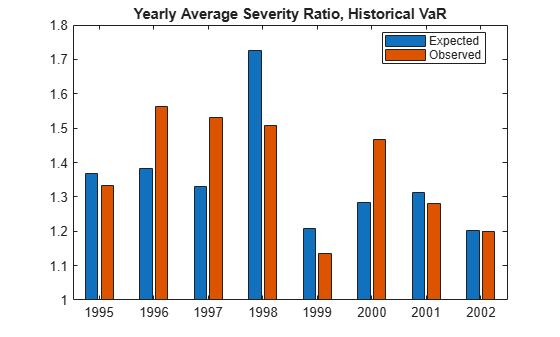However, a similar visualization with the expected vs. observed number of VaR failures shows that the `"Historical"` method tends to get violated many more times than expected. For example, even though in 2002 the expected average severity ratio is very close to the observed one, the number of VaR failures was more than twice the expected number. This then leads to test failures for both the unconditional normal and unconditional t tests.

```figure; subplot(2,1,1) plot(DatesTest,ReturnsTest,DatesTest,-VaR_Hist,DatesTest,-ES_Hist) hold on plot(DatesTest(FailureInd),ReturnsTest(FailureInd),'.') hold off legend('Returns','VaR','ES','Location','best') title('Historical VaR and ES') grid on subplot(2,1,2) bar(1995:2002,[sH.Expected,sH.Failures]) legend('Expected','Observed','Location','best') title('Yearly VaR Failures, Historical VaR')```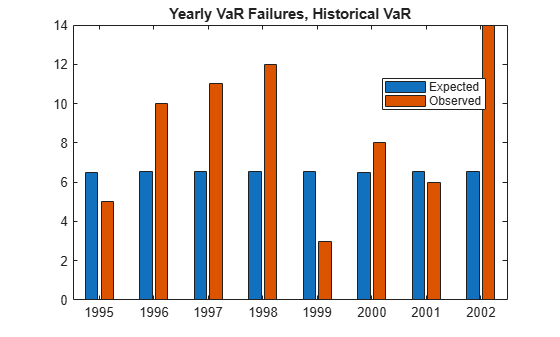### Simulation-Based Tests

The `esbacktestbysim` object supports three simulation-based ES back tests. `esbacktestbysim` requires the distribution information for the portfolio outcomes, namely, the distribution name (`"normal"` or `"t"`) and the distribution parameters for each day in the test window. `esbacktestbysim` uses the provided distribution information to run simulations to determine critical values. The tests supported in `esbacktestbysim` are `conditional`, `unconditional`, and `quantile`. These are implementations of the tests proposed in .

The `esbacktestbysim` object supports normal and t distributions. These tests can be used for any model where the underlying distribution of portfolio outcomes is normal or t, such as exponentially weighted moving average (EWMA), delta-gamma, or generalized autoregressive conditional heteroskedasticity (GARCH) models.

The `"Normal"`, `"T 10"`, and `"T 5"` models can be backtested with either the table-based tests in `esbacktest`, or with the simulation-based tests in `esbacktestbysim`. For illustration purposes, only `"T 5"` is backtested. The distribution name (`"t"`) and parameters (degrees of freedom, location, and scale) are provided when the `esbacktestbysim` object is created.

```rng('default'); % for reproducibility; the esbacktestbysim constructor runs a simulation ebts = esbacktestbysim(ReturnsTest,VaR_T5,ES_T5,"t",'DegreesOfFreedom',5,... 'Location',Mu,'Scale',SigmaT5,... 'PortfolioID',"S&P",'VaRID',"T 5",'VaRLevel',VaRLevel);```

The recommended workflow is the same: first, run the `summary` function, then run the `runtests` function, and then run the individual test functions. The `summary` function provides exactly the same information as the `summary` function from `esbacktest`.

```s = summary(ebts); disp(s)```
``` PortfolioID VaRID VaRLevel ObservedLevel ExpectedSeverity ObservedSeverity Observations Failures Expected Ratio Missing ___________ _____ ________ _____________ ________________ ________________ ____________ ________ ________ ______ _______ "S&P" "T 5" 0.975 0.97173 1.37 1.4075 2087 59 52.175 1.1308 0 ```

The `runtests` function shows the final accept or reject result.

```t = runtests(ebts); disp(t)```
``` PortfolioID VaRID VaRLevel Conditional Unconditional Quantile ___________ _____ ________ ___________ _____________ ________ "S&P" "T 5" 0.975 accept accept accept ```

Additional details on the test results are obtained by calling the individual test functions.

```[t,s] = conditional(ebts); disp(t)```
``` PortfolioID VaRID VaRLevel Conditional ConditionalOnly PValue TestStatistic CriticalValue VaRTest VaRTestResult VaRTestPValue Observations Scenarios TestLevel ___________ _____ ________ ___________ _______________ ______ _____________ _____________ _______ _____________ _____________ ____________ _________ _________ "S&P" "T 5" 0.975 accept accept 0.243 -0.027397 -0.084492 "pof" accept 0.34844 2087 1000 0.95 ```

The second output, `s`, contains simulated test statistic values assuming the distributional assumptions are correct. For example, `esbacktestbysim` generated 1000 scenarios of portfolio outcomes in this case, where each scenario is a series of 2087 observations simulated from t random variables with 5 degrees of freedom and the given location and scale parameters. The simulated values returned in the optional `s` output show typical values of the test statistic if the distributional assumptions are correct. These are the simulated statistics used to determine the significance of the tests, that is, the reported critical values and p-values.

`whos s`
``` Name Size Bytes Class Attributes s 1x1000 8000 double ```

The following figure is a visualization of the significance of the tests. The histogram shows the distribution of simulated test statistics, and the asterisk shows the value of the test statistic for the actual portfolio returns.

For the `conditional` test, this is a visualization of the standalone conditional test (`ConditionalOnly` result in the table above). The final conditional test result (`Conditional` column) depends also on a preliminary VaR backtest (`VaRTestResult` column).

```figure; histogram(s); hold on; plot(t.TestStatistic,0,'*'); hold off; Title = sprintf('Conditional: %s, p-value: %4.3f',t.VaRID,t.PValue); title(Title)```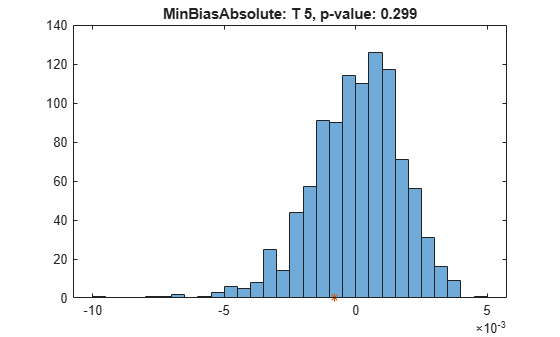Similar test details and visualizations can be obtained for the other tests. These are the `unconditional` test results. The unconditional test statistic reported by `esbacktestbysim` is exactly the same as the unconditional test statistic reported by `esbacktest`. However the critical values reported by `esbacktestbysim` are based on a simulation using a t distribution with 5 degrees of freedom and the given location and scale parameters. The `esbacktest` object gives approximate test results for the `"T 5"` model, whereas the results here are specific for the distribution information provided.

```[t,s] = unconditional(ebts); disp(t)```
``` PortfolioID VaRID VaRLevel Unconditional PValue TestStatistic CriticalValue Observations Scenarios TestLevel ___________ _____ ________ _____________ ______ _____________ _____________ ____________ _________ _________ "S&P" "T 5" 0.975 accept 0.13 -0.16179 -0.24268 2087 1000 0.95 ```
```figure; histogram(s); hold on; plot(t.TestStatistic,0,'*'); hold off; Title = sprintf('Unconditional: %s, p-value: %4.3f',t.VaRID,t.PValue); title(Title)```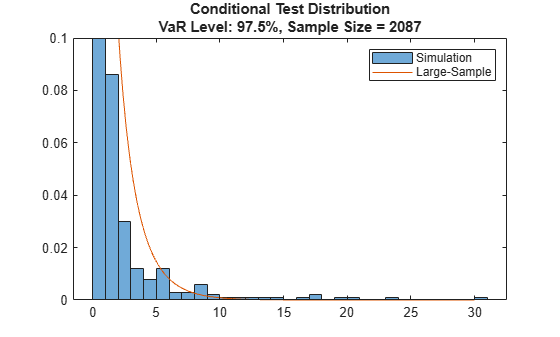Here are the results for the `quantile` test.

```[t,s] = quantile(ebts); disp(t)```
``` PortfolioID VaRID VaRLevel Quantile PValue TestStatistic CriticalValue Observations Scenarios TestLevel ___________ _____ ________ ________ ______ _____________ _____________ ____________ _________ _________ "S&P" "T 5" 0.975 accept 0.116 -0.067265 -0.095557 2087 1000 0.95 ```
```figure; histogram(s); hold on; plot(t.TestStatistic,0,'*'); hold off; Title = sprintf('Quantile: %s, p-value: %4.3f',t.VaRID,t.PValue); title(Title)```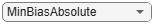The `"T 5"` model is accepted by the three tests.

The `esbacktestbysim` object provides a `simulate` function to run a new simulation. For example, if there is a borderline test result where the test statistic is near the critical value, you might use the `simulate` function to simulate new scenarios. In cases where more precision is required, a larger simulation can be run.

The `esbacktestbysim` tests can be run over a rolling window, following the same approach described above for `esbacktest`. User-defined traffic-light tests can also be defined, for example, using two different test confidence levels, similar to what was done above for `esbacktest`.

### Conclusions

To contrast the two ES backtesting objects:

• The `esbacktest` object can be used for a wider range of distributional assumptions (historical VaR, parametric VaR, Monte-Carlo VaR, extreme-value models). However, `esbacktest` offers approximate test results based on two variations of the same test: the unconditional test statistic with two different sets of precomputed critical values (`unconditionalNormal` and `unconditionalT`).

• The `esbacktestbysim` object can be used for parametric methods with normal and t distributions (which includes EWMA, GARCH, and delta-gamma). `esbacktestbysim` offers three different tests (`conditional`, `unconditional`, and `quantile`), and the critical values for these tests are simulated using the distribution information provided by the user, and are, therefore, more accurate.

As shown in this example, both ES backtesting objects provide functionality to generate reports on severities, VaR failures, and test results information. Both ES backtest objects provide the flexibility to build on them; for example, to create user-defined traffic-light tests, and to run the ES backtesting analysis over rolling windows.

### References

 Acerbi, C., and B. Szekely. "Backtesting Expected Shortfall." MSCI Inc., December 2014.

 Basel Committee on Banking Supervision. "Minimum Capital Requirements for Market Risk." January 2016, https://www.bis.org/bcbs/publ/d352.htm.

 McNeil, A., R. Frey, and P. Embrechts. Quantitative Risk Management: Concepts, Techniques and Tools. Princeton University Press. 2005.

 Rockafellar, R. T. and S. Uryasev. "Conditional Value-at-Risk for General Loss Distributions." Journal of Banking and Finance. Vol. 26, 2002, pp. 1443-1471.

### Local Functions

```function [VaR,ES] = hHistoricalVaRES(Sample,VaRLevel) % Compute historical VaR and ES % See  for technical details % Convert to losses Sample = -Sample; N = length(Sample); k = ceil(N*VaRLevel); z = sort(Sample); VaR = z(k); if k < N ES = ((k - N*VaRLevel)*z(k) + sum(z(k+1:N)))/(N*(1 - VaRLevel)); else ES = z(k); end end function [VaR,ES] = hNormalVaRES(Mu,Sigma,VaRLevel) % Compute VaR and ES for normal distribution % See  for technical details VaR = -1*(Mu-Sigma*norminv(VaRLevel)); ES = -1*(Mu-Sigma*normpdf(norminv(VaRLevel))./(1-VaRLevel)); end function [VaR,ES] = hTVaRES(DoF,Mu,Sigma,VaRLevel) % Compute VaR and ES for t location-scale distribution % See  for technical details VaR = -1*(Mu-Sigma*tinv(VaRLevel,DoF)); ES_StandardT = (tpdf(tinv(VaRLevel,DoF),DoF).*(DoF+tinv(VaRLevel,DoF).^2)./((1-VaRLevel).*(DoF-1))); ES = -1*(Mu-Sigma*ES_StandardT); end```

#### CECL and IFRS 9 Modeling in MATLAB: Measuring Lifetime Expected Credit Losses

Download white paper